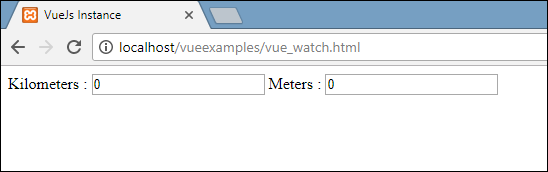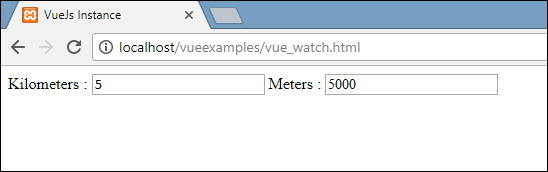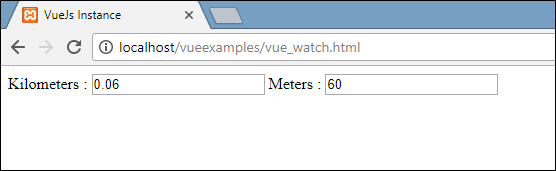# VueJS – Thuộc tính (tiếp theo)

In this chapter, we will learn about the Watch property. Using an example, we will see we can use the Watch property in VueJS.

## Example

```<html>
<head>
<title>VueJs Instance</title>
<script type = "text/javascript" src = "js/vue.js"></script>
</head>
<body>
<div id = "computed_props">
Kilometers : <input type = "text" v-model = "kilometers">
Meters : <input type = "text" v-model = "meters">
</div>
<script type = "text/javascript">
var vm = new Vue({
el: '#computed_props',
data: {
kilometers : 0,
meters:0
},
methods: {
},
computed :{
},
watch : {
kilometers:function(val) {
this.kilometers = val;
this.meters = val * 1000;
},
meters : function (val) {
this.kilometers = val/ 1000;
this.meters = val;
}
}
});
</script>
</body>
</html>```

In the above code, we have created two textboxes, one with kilometers and another with meters. In data property, the kilometers and meters are initialized to 0. There is a watch object created with two functions kilometers and meters. In both the functions, the conversion from kilometers to meters and from meters to kilometers is done.

As we enter values inside any of the texboxes, whichever is changed, Watch takes care of updating both the textboxes. We do not have to specially assign any events and wait for it to change and do the extra work of validating. Watch takes care of updating the textboxes with the calculation done in the respective functions.

Let’s take a look at the output in the browser.Let’s enter some values in the kilometers textbox and see it changing in the meters textbox and vice-versa.Let’s now enter in meters textbox and see it changing in the kilometers textbox. This is the display seen in the browser.